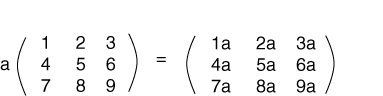Each element of the 3x3 result is 10.

# Multiplication of a Matrix by a Scalar

A matrix can be multiplied by a scalar (by a real number) as follows:

To multiply a matrix by a scalar, multiply each element of the matrix by the scalar.

Here is an example of this. (In this example, the variable a is a scalar.)### QUESTION 11:

Show the result if the scalar a in the above is the value -1.